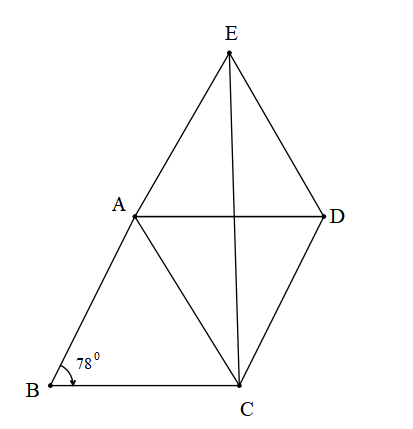QuestionAnswers

# ABCD is a rhombus and AED is an equilateral triangle. E and C lie on the opposite sides of AD. If $\angle ABC={{78}^{\circ }}$, calculate $\angle DCE$and $\angle ACE$.(a) ${{20}^{\circ }},{{30}^{\circ }}$(b) ${{21}^{\circ }},{{31}^{\circ }}$(c) ${{22}^{\circ }},{{32}^{\circ }}$(d) ${{19}^{\circ }},{{29}^{\circ }}$Hint: The key to solve the problem is to know the properties of the rhombus that opposite angles of rhombus are equal and diagonal of rhombus bisect the angles. Also, for this sum we need to know that all the angles of the equilateral triangle is ${{180}^{\circ }}$.

Complete step by step solution:
It is given that ABCD is a rhombus and AED is an equilateral triangle.
The angles of all equilateral triangles are ${{60}^{\circ }}$.
Therefore, $\angle ADE=\angle AED=\angle EAD={{60}^{\circ }}....................(i)$
Opposite angles of a rhombus are equal.
Therefore, $\angle ABC=\angle ADC={{78}^{\circ }}....................(ii)$
Also, all the sides of a rhombus are equal. By using this property ABC is an isosceles triangle with $AB=BC$.So the angles made by them will also be equal.
Therefore, $\angle BAC=\angle BCA...............(iii)$
By the property of the triangle that the sum of all the angles is ${{180}^{\circ }}$.
In ABC,
$\angle ABC+\angle BAC+\angle BCA={{180}^{\circ }}$
From equation (ii) and (iii), we get,
\begin{align} & {{78}^{\circ }}+\angle BAC+\angle BCA={{180}^{\circ }} \\ & 2\angle BAC={{102}^{\circ }} \\ & \angle BAC={{51}^{\circ }}...............(iv) \\ \end{align}
By the property that the diagonal of a rhombus is the angle bisector i.e. it divides the angle into two equal parts.
By using the above property, $\angle BCA=\angle ACD={{51}^{\circ }}................(v)$
From (i) and (ii), we know that $\angle EDC=\angle EDA+\angle ADC$
Therefore, $\angle EDC={{78}^{\circ }}+{{60}^{\circ }}={{138}^{\circ }}.......(vi)$
EDC is an isosceles triangle with $ED=DC$.So the angles made by them will also be equal.
Therefore, $\angle DEC=\angle DCE...............(vii)$
By the property of the triangle that the sum of all the angles is ${{180}^{\circ }}$.
In EDC,
$\angle EDC+\angle DEC+\angle DCE={{180}^{\circ }}$
From equation (vi) and (vii), we get,
\begin{align} & {{138}^{\circ }}+\angle DCE+\angle DCE={{180}^{\circ }} \\ & 2\angle DCE={{42}^{\circ }} \\ & \angle DCE={{21}^{\circ }}...............(viii) \\ \end{align}
From (v) and (viii), $\angle ACD={{51}^{\circ }}$and $\angle DCE={{21}^{\circ }}$
\begin{align} & \angle ACD=\angle ACE+\angle DCE \\ & {{51}^{\circ }}=\angle ACE+{{21}^{\circ }} \\ & \angle ACE={{51}^{\circ }}-{{21}^{\circ }}={{20}^{\circ }}..........................(ix) \\ \end{align}
Therefore, from (viii) and (ix) we get
$\angle DCE={{21}^{\circ }},\,\angle ACE={{20}^{\circ }}$ is the required solution.

Note: There is also an alternative way to find $\angle ACD$. We can also find by using the properties that the adjacent angles of rhombus sum up to ${{180}^{\circ }}$.
Therefore, $\angle ABC+\angle BCD={{180}^{\circ }}$
$\angle BCD={{180}^{\circ }}-{{78}^{\circ }}={{102}^{\circ }}$
By the property that the diagonal of a rhombus is the angle bisector i.e. it divides the angle into two equal parts.
By using the above property, $\angle ACD=\dfrac{\angle BCD}{2}=\dfrac{{{102}^{\circ }}}{2}={{51}^{\circ }}$.

View Notes
The Difference Between an Animal that is A Regulator and One that is A ConformerWhere There is a Will There is a Way EssayArea of a Rhombus FormulaLaughter is a Best Medicine EssayWhat is Syphilis?Area of Equilateral Triangle FormulaWhat is Polio?What is Photosynthesis?What is Soil?CBSE Class 10 Hindi A Question Paper 2020Hindi A Class 10 CBSE Question Paper 2009Hindi A Class 10 CBSE Question Paper 2015Hindi A Class 10 CBSE Question Paper 2016Hindi A Class 10 CBSE Question Paper 2012Hindi A Class 10 CBSE Question Paper 2010Hindi A Class 10 CBSE Question Paper 2007Hindi A Class 10 CBSE Question Paper 2013Hindi A Class 10 CBSE Question Paper 2008Hindi A Class 10 CBSE Question Paper 2014NCERT Solutions for Class 9 English Moments Chapter 8 - A House is Not a HomeNCERT Solutions Class 11 English Woven Words Essay Chapter 5 What is a Good BookNCERT Exemplar for Class 9 Science Chapter 2 - Is Matter Around Us Pure (Book Solutions)Lakhmir Singh Chemistry Class 9 Solutions Chapter 2 - Is Matter Around Us PureNCERT Solutions for Class 7 English An Alien Hand - A Tiger In The HouseNCERT Solutions for Class 9 Science Chapter 2 Is Matter Around Us Pure in HindiRS Aggarwal Class 9 Solutions Chapter-9 Congruence of Triangles and Inequalities in a TriangleNCERT Solutions for Class 9 Social Science Democratic Politics-I Chapter 1 - What is Democracy? Why Democracy?NCERT Solutions for Class 7 English An Alien Hand - Golu Grows A NoseNCERT Solutions Class 11 English Woven Words Poem Chapter 5 The World is too Much With Us